Thursday 19th May 2022# Classroom Key Stage 2 / KS2 - Maths Activities & Resources

## Welcome to the Maths section of the Key Stage 2 classroom. Within KS2 Maths you will find online @school assessment tests that will help children to enhance their learning and revise the subject of Maths at Key Stage 2 level.

•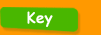•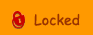•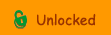••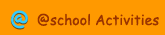•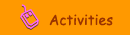•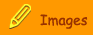The Activity you have selected is currently locked. Please register or Login to view.

###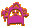Written Calculation Methods

Addition  compensation - An introduction to using the compensation method of addition in columns (Year 4-6)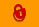Addition  counting on - Learn how to use informal written methods to carry out additions (Year 3)Addition in Columns  Introduction - An introduction to addition in columns. (Year 3)Addition in Columns 2 - An introduction to carrying when adding in columns (Year 4  6).Division 1 - An introduction to written methods for division (Year 4)Division 2 - An explanation of written methods for division (Year 5)Informal Subtraction  Counting Up - Learn how to use informal written methods to carry out subtractions (Year 3)Long Division - An explanation of written methods for long division (Year 6)Multiplication  Carrying - Introduction to carrying when multiplying in columns (Year 5/6)Multiplication  grid method - An introduction to the grid method when multiplying two-digit numbers (Year 4 )Multiplication  written methods - An introduction to the partition method when multiplying two-digit numbers (Year 4)Subtraction  compensation - Learn how to use informal written methods to carry out subtractions (Year 3)Subtraction  decomposition - An introduction to using the decomposition method of subtraction in columns (Year 3).Subtraction  decomposition 2 - Further progress with the decomposition method of subtraction in columns (Year 4  6).